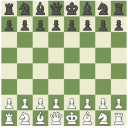# App Development

Announcements
Customer & Partners, DEC. 9, 11 AM ET: Qlik Product & Strategy Roadmap Session: Data Analytics REGISTER NOW
cancel
Showing results for
Did you mean:Employee

## interpret String as field: sum(total <Field> VALUE)

Hi folks,

I'm facing an issue of how to make the formula interpret a string as a field to be able to limit my calculations only to the selected value of this field.

Details:

There is a drill down dimension in a scatter plot. If user selects one bubble he then drills down to the next level seeing bubbles belonging to the selected bubble.

In this case the measure calculating sum(value) for these bubbles must calculate the % according to the following formula:

sum(VALUE) / sum(total <MyField> VALUE)

It works fine: if within <> I write MyField , the formula then looks the following way:  sum(VALUE) / sum(total VALUE)

PROBLEM: MyField variates due to the drilling down, so that it can be MyField1,MyField2,MyField3...

It does not work: if within <> I write MyVariable containing the needed field, the formula then looks the following way:  sum(VALUE) / sum(total VALUE)

It've tried out all possible combinations of how MyVariable should be and how do I use it within <> but all in vain.

Even this post did not help me: https://community.qlik.com/t5/QlikView-App-Development/Using-a-string-as-a-fieldname/td-p/1313243 (may be because my variable field lands within <>?).``Sum(VALUE) / Sum(TOTAL <\$(='[' & VariableNameHere & ']')> VALUE)``